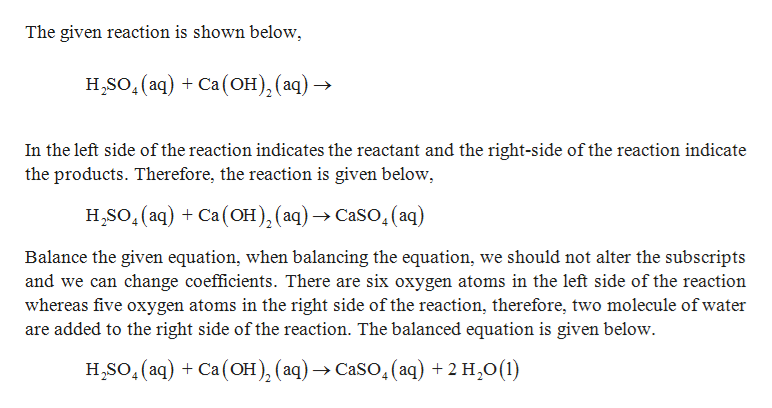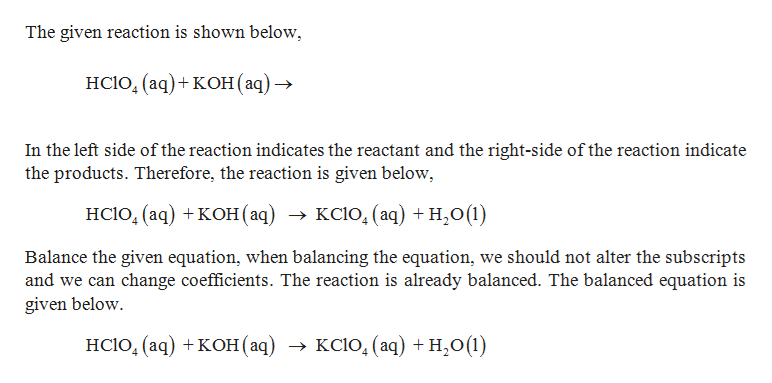# Complete and balance each of the following equations for acid-base reactions.H2SO4(aq)+Ca(OH)2(aq)→HClO4(aq)+KOH(aq)→H3PO4(aq)+NaOH(aq)→

Question
97 views

Complete and balance each of the following equations for acid-base reactions.

H2SO4(aq)+Ca(OH)2(aq)→

HClO4(aq)+KOH(aq)→

H3PO4(aq)+NaOH(aq)→

check_circle

Step 1

Balancing reaction:

Balanced reaction is a chemical reaction in which number of atoms for each element in the reaction and the total charge are same on both reactant side and the product side.

Steps in balancing the information

Step 1: Write the unbalanced equation

Step 2: Find the coefficient to balance the equation.

The coefficient should be reduced to the smallest whole number.help_outlineImage TranscriptioncloseThe given reaction is shown below H,SO(aq) Ca(OH), (aq) -» In the left side of the reaction indicates the reactant and the right-side of the reaction indicate the products. Therefore, the reaction is given below H,SO(aq)Ca(OH), (aq) ->CaSO.(aq) Balance the given equation, when balancing the equation, we should not alter the subscripts and we can change coefficients. There are six oxygen atoms in the left side of the reaction whereas five oxygen atoms in the right side of the reaction, therefore, two molecule of water are added to the right side of the reaction. The balanced equation is given below HSO(aq) Ca(OH), (aq)-> CaSO.(aq) +2 H20(1) fullscreen
Step 2

The given reaction ...help_outlineImage TranscriptioncloseThe given reaction is shown below HCIO, (aq) + кон (аq) > In the left side of the reaction indicates the reactant and the right-side of the reaction indicate the products. Therefore, the reaction is given below, HCIO, (aq) + кон (ад) > ксіо, (аq) + н,о(1) Balance the given equation, when balancing the equation, we should not alter the subscripts and we can change coefficients. The reaction is already balanced. The balanced equation is given below HCI0, (aq) + кон (аq) > ксіо, (аq) + н,о(1) fullscreen

### Want to see the full answer?

See Solution

#### Want to see this answer and more?

Solutions are written by subject experts who are available 24/7. Questions are typically answered within 1 hour.*

See Solution
*Response times may vary by subject and question.
Tagged in

### Chemistry